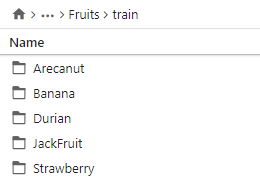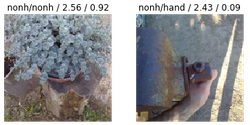# Inconsistency in interp.plot_top_losses

I have downloaded images from google images, Here is my folder structure. Please note that I just have a train folder and no valid and no test folders.After I train and try to plot losses (using `interp.plot_top_losses’), I get some inconsistent results. See below.

Can it be because I do not have a test and valid folders? Any thoughts?

1 Like

Remember that loss is not just accuracy, but confidence in the prediction. The text in `plot_top_losses` is `prediction/actual/loss/probability`. The cases you highlighted are ones where the model is making the correct prediction, but doing so with low confidence, leading to a higher loss.

4 Likes

Hi @krisho007,

Can you try following modified plot_losses function?

``````from fastai.callbacks.hooks import *
def plot_top_losses_heatmap(k,learner, largest= True,figsize=(15,11)):
tl_val,tl_idx = interp.top_losses(k,largest)
print (tl_idx)
classes = interp.data.classes
rows = math.ceil(math.sqrt(k))
fig,axes = plt.subplots(rows,rows,figsize=figsize)
fig.suptitle('prediction/actual/loss/probability', weight='bold', size=14)
for i,idx in enumerate(tl_idx):
im,cl = interp.data.valid_ds[idx]
cl = int(cl)
###
xb,_ = data.one_item(im)
xb = xb.cuda()
m = learner.model.eval()
with hook_output(m) as hook_a:
with hook_output(m, grad= True) as hook_g:
preds = m(xb)
preds[0,cl].backward()
acts = hook_a.stored.cpu()
avg_acts =acts.mean(0)
sz = im.shape[-1]
im.show(ax=axes.flat[i], title=
f'{classes[interp.pred_class[idx]]}/{classes[cl]} / {interp.losses[idx]:.2f} / {interp.probs[idx][cl]:.2f}')
axes.flat[i].imshow(avg_acts, alpha =0.6, extent= (0,sz,sz,0), interpolation='bilinear', cmap='magma')
``````

Then calling it

`plot_top_losses_heatmap(9,learn,True)`

I combined heatmaps with plot_loss , it may help you to see why are you getting inconsistent results.

It will highlight regions on image which learner thinks has more banana or Durian features (predicted class feature)

Very curious to see what it reveals in your case.

2 Likes

Now heatmap in incorporated in plot_top_losses function and by default its “on”.

I’m having a similar problem here; but the probability rates seem really high so I’m not sure why it’s showing the same class twice. Any ideas?

1 Like

Hey @asheinfeld, did you find an answer to this? I’m curious as to why this is happening!

It just mean there are very few misclassified examples. In this case only four, and when asking for 9 pictures, the fastai library pulls out the 5 correctly classified with the bigger loss e.g. the less confidence.

3 Likes

I think what @sgugger suggested below is the reason behind this. It makes sense to me now.

What is the probability of ?
in the first image 0.24 means its 24% confident out of all the other classes?
If so, that means the other classes confidence should be less than 0.24 ?
I am not able to understand the concept of probability

The latest version of fastai seems to have an issue with plot_top_losses().
Heatmap does not come up with
interp.plot_top_losses(9,figsize=(15,15),heatmap=True,heatmap_thresh=16)
I am running the notebook on colab.

Further Update:
Heatmap shows up at heatmap_thresh below 5.Actual value of heatmap_thresh does not have any bearing between 0 and 4. Further on some images there is no heatmap at all even though the classification is accurate with probability above 90%. How reliable is the heatmap to estimate whether the model is looking for relevant things in the image??

1 Like

As I have two classes I think that in my case a higher “confidence” should be accompanied by a lower loss, but this is not the case as you can see on the picture. Or is the loss function not the cross entropy by default?